Courses

# Fluid Dynamics MSQ

## 10 Questions MCQ Test Basic Physics for IIT JAM | Fluid Dynamics MSQ

Description
This mock test of Fluid Dynamics MSQ for Physics helps you for every Physics entrance exam. This contains 10 Multiple Choice Questions for Physics Fluid Dynamics MSQ (mcq) to study with solutions a complete question bank. The solved questions answers in this Fluid Dynamics MSQ quiz give you a good mix of easy questions and tough questions. Physics students definitely take this Fluid Dynamics MSQ exercise for a better result in the exam. You can find other Fluid Dynamics MSQ extra questions, long questions & short questions for Physics on EduRev as well by searching above.
*Multiple options can be correct
QUESTION: 1

### A massless conical flask filled with a liquid is kept on a table in a vaccum. The force exerted by the liquid on the base of the flask is W1. The force exerted by the flask on the table is W2.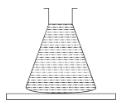Solution:

Pressure acts equally in all directions, and a liquid exerts forces on the walls of its container normal to the walls. Hence, the liquid forces on the sloping walls of the conical flask will have a net upward component.

The correct answers are: W1 > W2, The force exerted by the liquid on the walls of the flask is ((W1 – W2)

*Multiple options can be correct
QUESTION: 2

### A closed vessel in half filled with water. There is a hole near the top of the vessel and air is pumped out from this hole.

Solution:

The correct answers are: The pressure at the surface of the water will decrease, The force by the water on the bottom of the vessel will decrease

*Multiple options can be correct
QUESTION: 3

### A liquid of density ρ comes out with a velocity v from a horizontal tube of area of cross-section A. The reaction force exerted by the liquid on the tube is F.

Solution:

Volume of liquid discharged per second = v × 1 × A.
Mass of this liquid  = νAρ.
Momentum of this liquid = ν2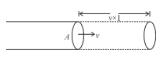The correct answers are: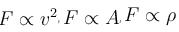*Multiple options can be correct
QUESTION: 4

Which of the following is not possible?

Solution:

The pressure at any point can never have different values.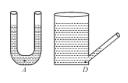In case of insufficient length of capillary tube the shape of meniscus is as below :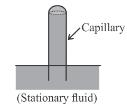*Multiple options can be correct
QUESTION: 5

A cubical box is completely filled with mass m of a liquid and is given horizontal acceleration a as shown. Match the force due to fluid pressure on the faces of the cube with their appropriate values (assume zero pressure at minimum pressure)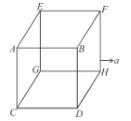Solution:

Pressure varies with height ⇒ P = ρgh

and is horizontal with acceleration ⇒ P = ρℓa

So on (A) ρgh part is zero while average of ρax is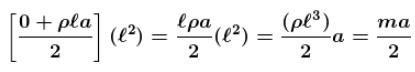In (B) pℓa part is zero while average of pgx is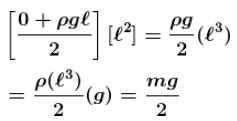Similarly for other part.

The correct answers are: force on face ABFE is mg/2, force on face BFHD is mg/2, , force on face CGHD is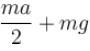*Multiple options can be correct
QUESTION: 6

A cuboid is filled of density ρ2 upto height and with liquid of density ρ2, also upto height h as shown. Which of the following are true :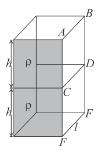Solution:

On ABCD avg pressure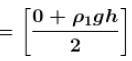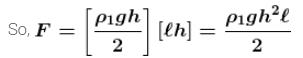No. of contact of ρ2 and not any pressure on ABCD due to ρ2gh

On CDEF due to ρ1, at every point pressure is ρ1gh so average is also ρ1gh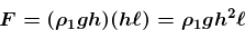On CDEF due to ρ1 constant but ρ1 is variable so average is ρ1 will be taken.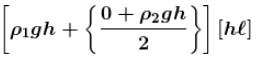The correct answers are: Force on face ABCD due to liquid of density ρ1 is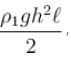Force on face CDEF due to liquid of density ρ1 is ρ1gh2ℓ, Force on face CDEF due to liquid of density ρ2 is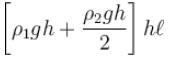*Multiple options can be correct
QUESTION: 7

A cylindrical vessel of 90 cm height is kept filled the brim. It has four holes 1, 2, 3, 4 which are respectively at heights of 20cm, 30 cm, 40 cm and 50 cm from the horizontal floor PQ. The water falling at the maximum horizontal distance from the vessel comes from :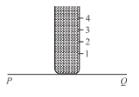Solution:

The maximum horizontal distance from the vessel comes from hole number 3 and 4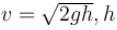is height of hole from top.
horizontal distance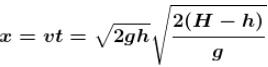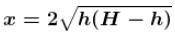The correct answers are: hole number 4, hole number 3

*Multiple options can be correct
QUESTION: 8

A tank, which is open at the top, contains a liquid up to a height H. A small hole is made in the side of the tank at a distance y below the liquid surface. The liquid emerging from the hole lands at a distance x from the tank.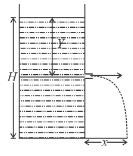Solution:

The velocity of efflux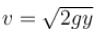The emerging liquid moves as a projectile and reaches the ground in time t, where,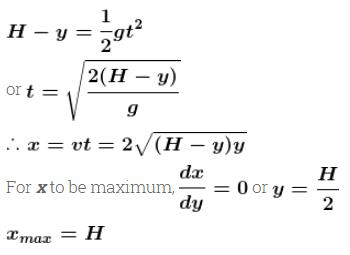The correct answers are: If y is increased from zero to Hx will first increase and then decrease, x is maximum for y = H/2, The maximum value of x is H.

*Multiple options can be correct
QUESTION: 9

There is a small hole near the bottom of an open tank filled with a liquid. The speed of the water ejected does not depend on :

Solution:

The correct answers are: area of the hole, density of the liquid

*Multiple options can be correct
QUESTION: 10

The vessel shown in the figure has two sections of areas of cross-section A1 and A2. A liquid of density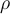fills both the sections, up to a height h in each. Neglect atmospheric pressure. Then which of the following are correct.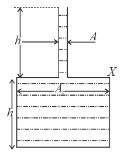Solution:

The correct answers are: The pressure at the base of the vessel is 2hρg. , The force exerted by the liquid on the base of the vessel is 2hρgA2. , The weight of the liquid is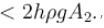, The walls of the vessel at the level X exert a downward force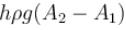on the liquid.